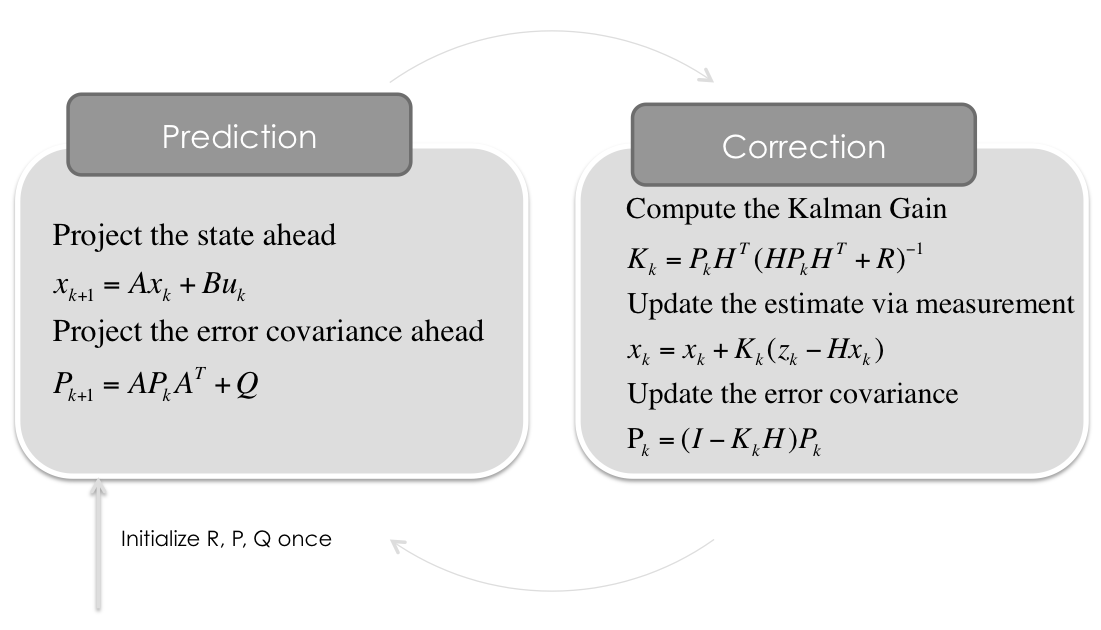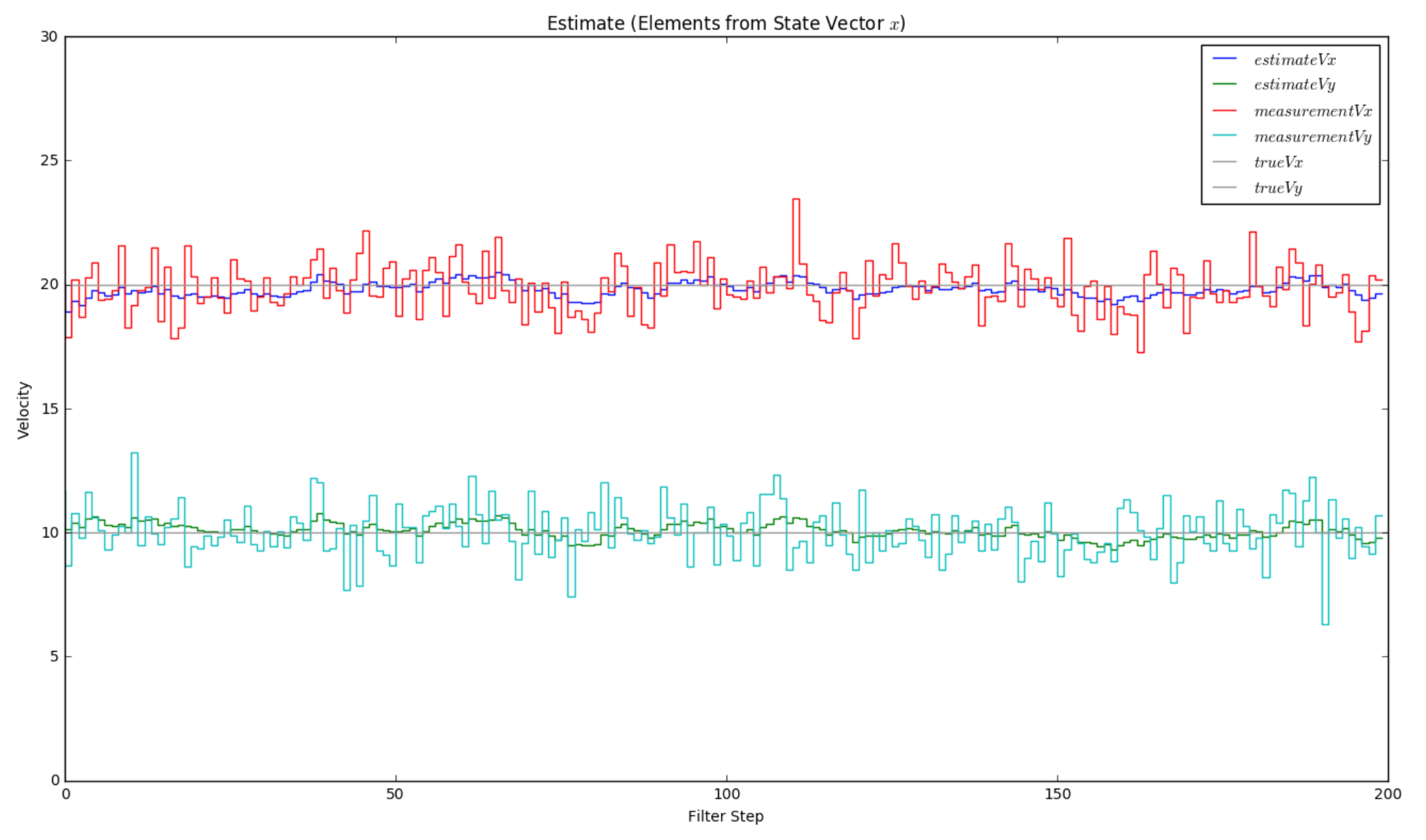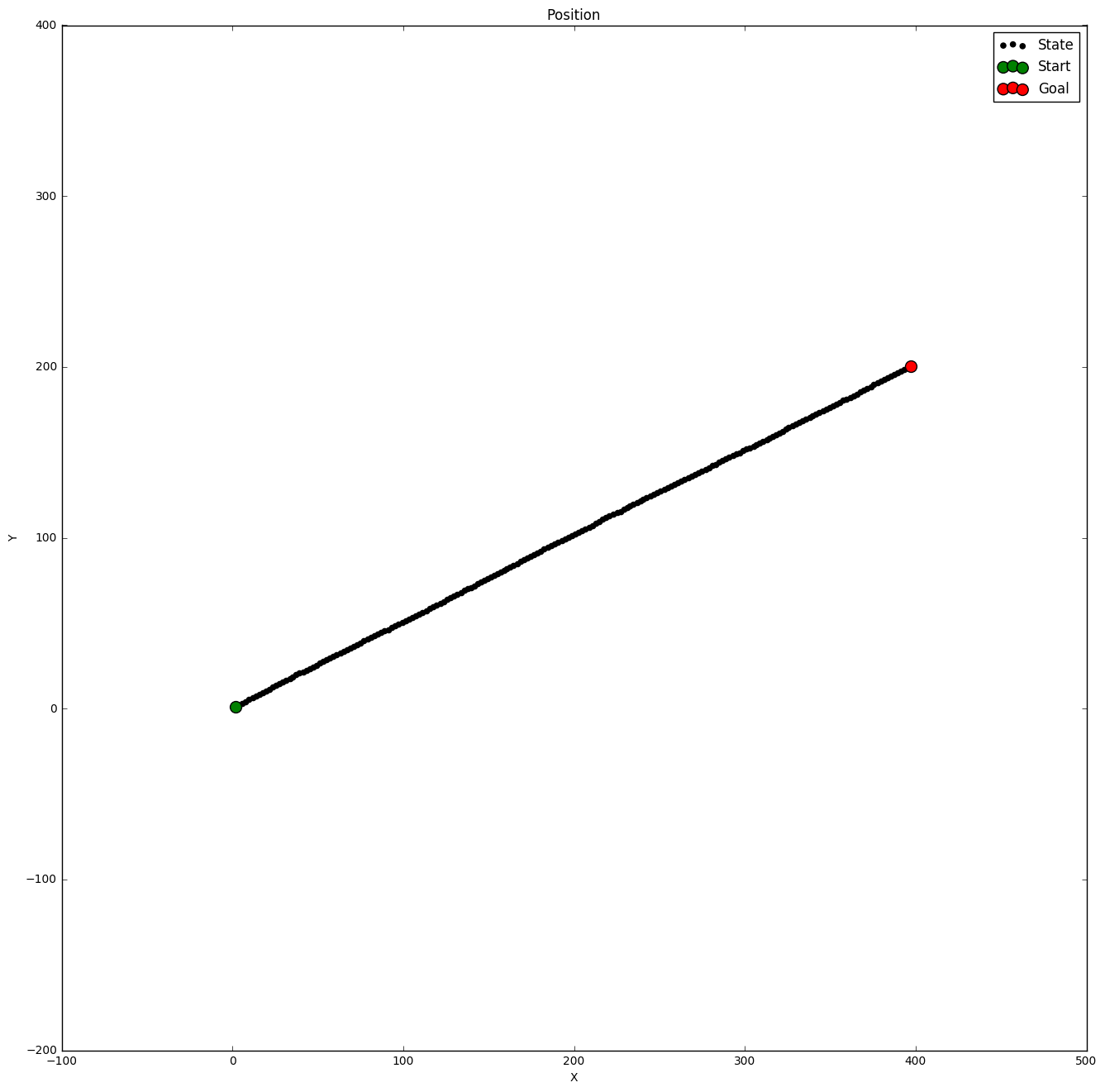《无人驾驶》是由张杨执导，刘烨、高圆圆、李小冉、陈建斌、林心如、王珞丹、黄轩等主演的都市爱情电影。影片讲述了数对都市男女之间的爱情纠葛，一场撞车将他们的命运纠缠在了一起。   该片于2010年7月2日在中国内地上映。 展开全文《无人驾驶》是由张杨执导，刘烨、高圆圆、李小冉、陈建斌、林心如、王珞丹、黄轩等主演的都市爱情电影。影片讲述了数对都市男女之间的爱情纠葛，一场撞车将他们的命运纠缠在了一起。   该片于2010年7月2日在中国内地上映。

Driverless

105分钟

2010年7月2日  中国

Spacked out

• 无人驾驶汽车是智能汽车的一种，也称为轮式移动机器人，主要依靠车内的以计算机系统为主的智能驾驶仪来实现无人驾驶的目标。无人驾驶汽车是通过车载传感系统感知道路环境，自动规划行车路线并控制车辆到达预定目标的...
无人驾驶汽车是智能汽车的一种，也称为轮式移动机器人，主要依靠车内的以计算机系统为主的智能驾驶仪来实现无人驾驶的目标。无人驾驶汽车是通过车载传感系统感知道路环境，自动规划行车路线并控制车辆到达预定目标的智能汽车。  它是利用车载传感器来感知车辆周围环境，并根据感知所获得的道路、车辆位置和障碍物信息，控制车辆的转向和速度，从而使车辆能够安全、可靠地在道路上行驶。  集自动控制、体系结构、人工智能、视觉计算等众多技术于一体，是计算机科学、模式识别和智能控制技术高度发展的产物，也是衡量一个国家科研实力和工业水平的一个重要标志，在国防和国民经济领域具有广阔的应用前景
展开全文传感器 人工智能 智能汽车
• 前言：随着深度学习近几年来的突破性进展，无人驾驶汽车也在这些年开始不断向商用化推进。很显然，无人驾驶汽车已经不是遥不可及的“未来技术”了，未来10年必将成为一个巨大的市场。本系列博客将围绕当前使用的最...

前言：随着深度学习近几年来的突破性进展，无人驾驶汽车也在这些年开始不断向商用化推进。很显然，无人驾驶汽车已经不是遥不可及的“未来技术”了，未来10年必将成为一个巨大的市场。本系列博客将围绕当前使用的最先进的无人驾驶汽车相关技术，一步一步地带领大家学习并且掌握无人驾驶系统的每一个模块的理论基础和实现细节。由于无人驾驶汽车系统构成及其复杂，本系列博客仅讨论软件部分的内容，关于汽车，传感器和底层的硬件，不是我们关注的重点。至于连载的顺序和技术路线，本人其实是同时开始的，先完成哪一节就先发那一节。

首先我将带大家了解无人驾驶汽车系统感知模块的重要技术——卡尔曼滤波，卡尔曼滤波部分我打算分三节（三次博客的内容）：
卡尔曼滤波与行人状态估计扩展卡尔曼滤波（EKF）与传感器融合过程模型，无损卡尔曼滤波（UKF）与车辆状态轨迹
本节为卡尔曼滤波，主要讲解卡尔曼滤波的具体推导，卡尔曼滤波在行人状态估计中的一个小例子。
为什么要学卡尔曼滤波？
卡尔曼滤波以及其扩展算法能够应用于目标状态估计，如果这个目标是行人，那么就是行人状态估计（或者说行人追踪），如果这个目标是自身，那么就是车辆自身的追踪（结合一些地图的先验，GPS等数据的话就是自身的定位）。在很多的无人驾驶汽车项目中，都能找到卡尔曼滤波的扩展算法的身影（比如说EKF，UKF等等）。本节我们从最简单的卡尔曼滤波出发，完整的理解一遍卡尔曼滤波的推导过程，并实现一个简单的状态估计Python程序。
卡尔曼滤波是什么？
我们通常要对一些事物的状态去做估计，为什么要做估计呢？因为我们通常无法精确的知道物体当前的状态。为了估计一个事物的状态，我们往往会去测量它，但是我们不能完全相信我们的测量，因为我们的测量是不精准的，它往往会存在一定的噪声，这个时候我们就要去估计我们的状态。卡尔曼滤波就是一种结合预测（先验分布）和测量更新（似然）的状态估计算法。

一些概率论的知识基础

下面是一些概率论的基础知识，如果之前有这方面的知识储备那当然是最好的，很有利于我们理解整个博客内容，如果没有这方面的基础而且也看不懂下面的内容也没关系，我会以一个相对直观的方式来展现整个理论部分。

先验概率

P

(

X

)

P(X)

：仅仅依赖主观上的经验，事先根据已有的只是的推断

后验概率

P

(

X

∣

Z

)

P(X|Z)

：是在相关证据或者背景给定并纳入考虑以后的条件概率似然

P

(

Z

∣

X

)

P(Z|X)

：已知结果区推测固有性质的可能性

贝叶斯公式：

P

(

A

∣

B

)

=

P

(

A

)

×

P

(

B

∣

A

)

P

(

B

)

P(A|B) = \frac{P(A) \times P(B|A)}{P(B)}

后验分布正比于先验分布乘以似然。

卡尔曼滤波完整推导
一个简单的例子
若干年后，我们的可回收火箭要降落到地球，我们比较关心的状态就是我们的飞行器的高度了，飞行器的高度就是我们想要估计的状态，我们会通过一些传感器去测量我们当前的高度信息，比如说使用气压计。假如我们每一次测量，当前高度都变成上一次测量的

95

%

95\%

，那么我们就可以得到如下关系：

H

e

i

g

h

t

(

t

)

=

0.95

×

H

e

i

g

h

t

(

t

−

1

)

Height^{(t)} = 0.95 \times Height^{(t-1)}

我们可以使用递归来表示这样的公式——为了计算我们当前的高度，我们必须知道我们上一个测量的高度，以此递推，我们就会推到我们的飞行器的初始高度。
但是，由于我们的测量往往来自于一些传感器（比如说GPS，气压计），所以测量的结果总是带有噪声的，这个噪声是有传感器本身引起的，那么我们的表达式就变成了：

M

e

a

s

u

r

e

m

e

n

t

(

t

)

=

H

e

i

g

h

t

(

t

)

+

N

o

i

s

e

(

t

)

Measurement^{(t)} = Height^{(t)} + Noise^{(t)}

这个噪声我们称之为测量噪声 (

M

e

a

s

u

r

e

m

e

n

t

Measurement

N

o

i

s

e

Noise

)。通常来说，这种噪声都满足高斯分布。我们用符号描述以上两部分：

x

k

=

a

x

k

−

1

x_k = a x_{k-1}

z

k

=

x

k

+

v

k

z_k = x_k + v_k

第一个式子是我们的 过程模型，是我们的经验（比如说一些运动模型，牛顿力学等等），我们用这种过程模型去 预测 我们考察的事物的状态（在没有任何信息的情况下，或者说在没有任何测量数据的情况下）；第二个式子是测量的表达式，它大致描述了我们测量的组成成分，我们用这个测量去 更新 我们对状态的估计。 其中

x

k

x_k

是我们的飞行器当前的状态，

x

k

−

1

x_{k-1}

是我们上一个状态（注意状态和测量的区别），

z

k

z_k

使我们当前对飞行器的测量，

v

k

v_k

使我们当前的测量噪声，

a

a

是一个常数，在我们这个例子里面就是

0.95

0.95

。

思考一下，在这里我们用一个简单的比例（常数）来描述“可回收火箭”的运动规律，会存在什么问题？

很显然，现实中的运动不会像我们这个简单的过程模型一样高度按比例缩小。在这里为了简化，我们先假设我们的模型挺好的，能够大致描述出这颗“神奇火箭”的运动规律，只是偶尔会存在一定的偏差（比如所空气湍流的影响），那么我们在计算

x

k

x_k

时在加一个噪声来描述我们的过程模型与实际运动的差异，这个噪声我们称之为过程噪声 (

P

r

o

c

e

s

s

Process

N

o

i

s

e

Noise

)，我们用

w

t

w_t

来表示这种噪声，那么

x

k

x_k

的计算公式就变成：

x

k

=

a

x

k

−

1

+

w

k

x_k = a x_{k-1} + w_k

为了简化，后面的分析我们会先忽略过程噪声，但是我们在传感器融合的部分会将过程噪声重新考虑进来。
状态估计
因为我们是要估计飞行器的状态（高度），所以我们把测量的公式变换成：

x

k

=

z

k

−

v

k

x_k =z_k - v_k

显然，在这里

v

k

v_k

是没办法知道的，卡尔曼滤波通过同时考虑上一状态值和当前的测量值来获得对当前状态值的估计。一般我们用

x

^

\hat{x}

来表示对状态

x

x

的估计。那么

x

^

k

\hat{x}_k

就表示当前状态的估计。下面我们可以用如下公式来描述卡尔曼滤波如何结合上一个估计和和现在的测量来产生对当前的估计：

x

^

k

=

x

^

k

−

1

+

g

k

(

z

k

−

x

^

k

−

1

)

\hat{x}_k = \hat{x}_{k-1} + g_k(z_k - \hat{x}_{k-1})

这里的

g

k

g_k

叫做 卡尔曼增益（Kalman Gain），它描述的是之前的估计和当前的测量对当前的估计的影响的分配权重。为了理解，我们考虑极端的例子，如果

g

k

=

0

g_k=0

，也就是说增益为

0

0

，那么：

x

^

k

=

x

^

k

−

1

\hat{x}_k = \hat{x}_{k-1}

也就是说我们非常不信任我们当前的测量，我们直接保留了我们上一次的估计作为我们对当前状态的估计。如果

g

k

=

1

g_k=1

，即增益为

1

1

，则：

x

^

k

=

z

k

\hat{x}_k = z_{k}

即我认为当前的测量非常可信，我彻底接受它作为我当前状态的估计。那么当

g

k

g_k

介于

(

0

,

1

)

(0, 1)

，则表示对两者的权重分配。
计算卡尔曼增益
那么如何计算卡尔曼增益呢？我们使用一种间接的方法，我们虽然不知道测量噪声

v

k

v_k

的值，但是我们知道它的均值，前面我们提到，测量噪声来自传感器本身，并且符合高斯分布，所以我们能够从传感器厂商那里获得测量噪声的均值

r

r

，那么

g

k

g_k

可以表示为：

g

k

=

p

k

−

1

/

(

p

k

−

1

+

r

)

g_k = p_{k-1}/(p_{k-1} + r)

其中

p

k

p_k

叫预测误差，表达式为：

p

k

=

(

1

−

g

k

)

p

k

−

1

p_k = (1 - g_k)p_{k-1}

那么怎么理解

g

k

g_k

和

p

k

p_k

呢？
那么假设前一次的预测误差

p

k

−

1

=

0

p_{k-1} = 0

， 那么根据公式，当前的增益

g

k

=

0

g_k = 0

，一维着舍弃掉当前的测量而完全采用上一个时刻的估计，如果

p

k

−

1

=

1

p_{k-1} = 1

那么增益变成

1

/

(

1

+

r

)

1 / (1 + r)

通常

r

r

是个很小的数值，所以增益为1，所以完全接受这一次的测量作为我们的估计（因为上一次的的预测误差太大了，为1，所以一旦拿到了新的测量，如获至宝，就干脆把不准确的上次的估计舍弃掉了）
对于下面的公式的分析是一样的，我们考虑极端的例子，当增益为

0

0

，

p

k

=

p

k

−

1

p_k = p_{k-1}

，因为我们彻底舍弃掉了本次的测量，所以本次的预测误差只能接受上一次的。当增益为

1

1

，

p

k

=

0

p_k = 0

。
预测和更新
那么现在我们有关于当前的火箭高度状态的两个公式了，它们分别是：

x

k

=

a

x

k

−

1

x_k = a x_{k-1}

和

x

^

k

=

x

^

k

−

1

+

g

k

(

z

k

−

x

^

k

−

1

)

\hat{x}_k = \hat{x}_{k-1} + g_k(z_k - \hat{x}_{k-1})

那么我们到底要用哪一个呢？答案是我们都用，第一个公式我们称之为预测，是基于一些先验的知识（比如说运动模型，牛顿力学等等）觉得我们的状态应该是这样的，而第二个公式呢，就是我们基于我们“不完美的”的传感器的测量数据来更新我们对状态的估计。另为，预测，理论上只考虑了一个固定的过程模型和过程噪声，但是由于我们现在是对机械的状态进行估计，在预测过程中需要对机械本身的控制建模， 我们在预测部分再新增一个控制信号，我们用

b

u

k

bu_k

表示。实际的传感器测量除了会有测量噪声

v

k

v_k

以外，还会存在一定的关于真实状态的缩放，因此我们使用

x

k

x_k

表示测量时通常还会在其前面加一个缩放系数

c

c

。 结合这写我们就可以得到卡尔曼滤波预测和更新过程了：
预测

x

^

k

=

a

x

^

k

−

1

+

b

u

k

\hat{x}_k = a \hat{x}_{k-1} + b u_k

p

k

=

a

p

k

−

1

a

p_k = a p_{k-1} a

卡尔曼滤波更新的过程为：

g

k

=

p

k

c

/

(

c

p

k

c

+

r

)

g_k = p_k c / (c p_k c + r)

x

^

k

←

x

^

k

+

g

k

(

z

k

−

c

x

^

k

)

\hat{x}_k \leftarrow \hat{x}_k + g_k(z_k - c\hat{x}_k)

p

k

←

(

1

−

g

k

c

)

p

k

p_k \leftarrow (1 - g_k c)p_k

使用线性代数的方法来表示预测和更新
预测

x

^

k

=

A

x

^

k

−

1

+

B

u

k

\hat{x}_k = A \hat{x}_{k-1} + B u_k

P

k

=

A

P

k

−

1

A

T

P_k = A P_{k-1} A^T

卡尔曼滤波更新的过程为：

G

k

=

P

k

C

T

(

C

P

k

C

T

+

R

)

−

1

G_k = P_k C^T (C P_k C^T + R)^{-1}

x

^

k

←

x

^

k

+

G

k

(

z

k

−

C

x

^

k

)

\hat{x}_k \leftarrow \hat{x}_k + G_k(z_k - C\hat{x}_k)

P

k

←

(

1

−

G

k

C

)

P

k

P_k \leftarrow (1 - G_k C)P_k

至此，卡尔曼滤波的完整推导就结束了。下面，我们来看看卡尔曼滤波在无人汽车的感知模块的应用。

卡尔曼滤波算法为什么会叫滤波算法？ 以一维卡尔曼滤波为例，如果我们单纯的相信测量的信号，那么这个信号是包含噪声的，是很毛糙的，但是当我们运行卡尔曼滤波算法去做估计，我们估计的信号会很光滑，看起来似乎滤掉了噪声的影响，所以称之为滤波算法。实际上，卡尔曼滤波不仅仅过滤掉了测量信号的噪声，它同时也结合了以往的估计，卡尔曼滤波在线性问题中被证明是最优估计。

卡尔曼滤波在无人驾驶汽车感知模块的应用
无人车感知模块的传感器
无人驾驶汽车要安全的在道路上行驶，需要“耳听六路，眼观八方”。那么无人车的耳朵和眼睛是什么呢？那就是安装在无人车上的各种各样的传感器了。无人车上的传感器能够多达几十个，而且是不同种类的，比如：
立体摄像机往往用于获取图像和距离信息；交通标志摄像机用于交通标志的识别；雷达一般安装在车辆的前保险杆里面，用于测量相对于车辆坐标系下的物体，可以用来定位，测距，测速等等，容易受强反射物体的干扰，通常不用于静止物体的检测；激光雷达往往安装在车顶，使用红外激光束来获得物体的距离和位置，但是空间分辨率高，但是笨重，容易被天气影响。
由此可知，各种传感器都有其优点和缺点，在实际的无人驾驶汽车里，我们往往结合多种传感器的数据去感知我们的车辆周边的环境。这种结合各种传感器的测量数据的过程我们称之为传感器融合（Sensor Fusion）。本系列博客后面的章节我将详细给大家介绍基于扩展卡尔曼滤波和无损卡尔曼滤波在传感器融合中的应用。在本节中，我们主要考虑卡尔曼滤波基于单一的传感器数据来估算行人位置的方法。
基于卡尔曼滤波的行人位置估算
卡尔曼滤波虽然简单，确实无人驾驶汽车的技术树中非常重要的一部分，当然，在真实的无人驾驶汽车项目中使用到的技术是相对更加复杂的，但是其基本原理仍然是本博客介绍的这些内容。在无人驾驶中，卡尔曼滤波主要可以用于一些状态的估计，主要应用于行人，自行车以及其他汽车的状态估计。下面，我们以行人的状态估计为例展开。
当我们要估计一个行人的状态时，首先就是要建立起腰估计的状态的表示。这里，行人的状态大致可以表示为

x

=

(

p

,

v

)

x=(p, v)

， 其中

p

p

为行人的位置，而

v

v

则是行人此时的速度。我们用一个向量来表示一个状态：

x

=

(

p

x

,

p

y

,

v

x

,

v

y

)

T

x = (p_x, p_y, v_x, v_y)^T

在确定了我们要估计的状态以后，我们需要确定一个过程模型，如前文所说，过程模型用于预测阶段来产生一个对当前估计的先验。在本文中，我们先以一个最简单的过程模型来描述行人的运动——恒定速度模型：

x

k

+

1

=

A

x

k

+

ν

x_{k+1} = A x_{k} + \nu

即：之所以称之为恒定速度模型，是因为我们将上面这个行列式展开可以得到：

p

x

k

+

1

=

p

x

k

+

v

x

k

Δ

t

p_x^{k+1} = p_x^{k} + v_x^{k} \Delta t

p

y

k

+

1

=

p

y

k

+

v

y

k

Δ

t

p_y^{k+1} = p_y^{k} + v_y^{k} \Delta t

v

x

k

+

1

=

v

x

k

v_x^{k+1} = v_x^{k}

v

y

k

+

1

=

v

y

k

v_y^{k+1} = v_y^{k}

恒定速度过程模型假定预测的目标的运动规律是恒定的速度的，在行人状态预测这个问题中，很显然行人并不一定会以恒定的速度运动，所以我们的过程模型包含了一定的 过程噪声，在这个问题中过程噪声也被考虑了进来，其中的

ν

\nu

是我们这个过程模型的过程噪声。在行人状态估计中的过程噪声其实就是行人的加减速，那么我们原来的处理过程就变成了：我们预测的第二步就变成了：

A

′

​

​

=

A

P

A

T

+

Q

A′​​ =APA^T+Q

这是我们的

A

A

的更新过程，它本质上是我们的估计状态概率分布的协方差矩阵。

Q

Q

是我们的过程噪声的协方差矩阵，由于过程噪声是随机带入的，所以

ν

\nu

本质是一个高斯分布：

ν

∼

N

(

0

,

Q

)

\nu \thicksim N(0, Q)

，Q是过程噪声的协方差，Q的形式为：进而表示为：

Q

=

G

⋅

G

T

⋅

σ

v

2

Q = G\cdot G^T \cdot \sigma_v^2

其中

G

=

[

0.5

Δ

t

2

,

0.5

Δ

t

2

,

Δ

t

,

Δ

t

]

T

G={[0.5 \Delta t^2, 0.5 \Delta t^2, \Delta t, \Delta t]}^T

,

σ

v

2

\sigma_v^2

对于行人我们可以设置为

0.5

m

/

s

2

0.5 m/s^2

（这一部分大家如果想要详细了解可以阅读这篇文章：http://ieeexplore.ieee.org/xpl/articleDetails.jsp?arnumber=5940526）。
测量步骤中，我们直接可以测量到速度

v

x

v_x

和

v

y

v_y

，所以我们的测量矩阵

C

C

可以表示为：测量噪声的协方差矩阵

R

R

为：其中的 $\sigma^2_{v_x}$ 和

s

i

g

m

a

v

x

2

sigma^2_{v_x}

描述了我们的传感器的测量能够有多“差”，这是传感器固有的性质，所以往往是传感器厂商提供。
最后，在测量的最后一步，我们使用单位矩阵

I

I

来代替原式中的1:

P

k

←

(

I

−

G

k

C

)

P

k

P_k \leftarrow (I - G_k C)P_k

至此，基于恒定速度过程模型卡尔曼滤波的行人状态估计的整个流程我们就讲完了，下面我们使用Python将整个过程实现一下。
卡尔曼滤波行人状态估计Python例子
首先我们看一下卡尔曼滤波的整个流程，其实在实际的论文和资料中，预测矩阵通常使用

F

F

来表示（我们前文中一直是使用

A

A

）,测量矩阵通常使用

H

H

表示（我们前文中使用的是

C

C

）,卡尔曼增益通常使用

K

K

来表示（我们前文中使用的是

G

G

）,下面是文献材料中通常意义的卡尔曼滤波过程：注意：公式还包含一项：

B

μ

B \mu

,这一项是指我们在追踪一个物体的状态的时候把它内部的控制也考虑进去了，这在行人，自行车，其他汽车的状态估计问题中是无法测量的，所以在这个问题中我们设置

B

μ

B \mu

为

0

0

.

我们的代码将在IPython中执行，大家可以中jupyter notebook交互式地运行。
首先我们载入必要的库：
import numpy as np
%matplotlib inline
import matplotlib.pyplot as plt
from scipy.stats import norm

接着我们初始化行人状态x, 行人的不确定性（协方差矩阵）P，测量的时间间隔dt，处理矩阵F以及测量矩阵H：
x = np.matrix([[0.0, 0.0, 0.0, 0.0]]).T
print(x, x.shape)
P = np.diag([1000.0, 1000.0, 1000.0, 1000.0])
print(P, P.shape)

dt = 0.1 # Time Step between Filter Steps
F = np.matrix([[1.0, 0.0, dt, 0.0],
[0.0, 1.0, 0.0, dt],
[0.0, 0.0, 1.0, 0.0],
[0.0, 0.0, 0.0, 1.0]])
print(F, F.shape)

H = np.matrix([[0.0, 0.0, 1.0, 0.0],
[0.0, 0.0, 0.0, 1.0]])
print(H, H.shape)

ra = 10.0**2

R = np.matrix([[ra, 0.0],
[0.0, ra]])
print(R, R.shape)

计算测量过程的噪声的协方差矩阵R和过程噪声的协方差矩阵Q：

ra = 0.09
R = np.matrix([[ra, 0.0],
[0.0, ra]])
print(R, R.shape)

sv = 0.5
G = np.matrix([[0.5*dt**2],
[0.5*dt**2],
[dt],
[dt]])
Q = G*G.T*sv**2
from sympy import Symbol, Matrix
from sympy.interactive import printing
printing.init_printing()
dts = Symbol('dt')
Qs = Matrix([[0.5*dts**2],[0.5*dts**2],[dts],[dts]])
Qs*Qs.T


定义一个单位矩阵：
I = np.eye(4)
print(I, I.shape)

我们随机产生一些测量数据：
m = 200 # Measurements
vx= 20 # in X
vy= 10 # in Y

mx = np.array(vx+np.random.randn(m))
my = np.array(vy+np.random.randn(m))
measurements = np.vstack((mx,my))

print(measurements.shape)
print('Standard Deviation of Acceleration Measurements=%.2f' % np.std(mx))
print('You assumed %.2f in R.' % R[0,0])

fig = plt.figure(figsize=(16,5))
plt.step(range(m),mx, label='$\dot x$')
plt.step(range(m),my, label='$\dot y$')
plt.ylabel(r'Velocity $m/s$')
plt.title('Measurements')
plt.legend(loc='best',prop={'size':18})

一些过程值，用于结果的显示：
xt = []
yt = []
dxt= []
dyt= []
Zx = []
Zy = []
Px = []
Py = []
Pdx= []
Pdy= []
Rdx= []
Rdy= []
Kx = []
Ky = []
Kdx= []
Kdy= []

def savestates(x, Z, P, R, K):
xt.append(float(x))
yt.append(float(x))
dxt.append(float(x))
dyt.append(float(x))
Zx.append(float(Z))
Zy.append(float(Z))
Px.append(float(P[0,0]))
Py.append(float(P[1,1]))
Pdx.append(float(P[2,2]))
Pdy.append(float(P[3,3]))
Rdx.append(float(R[0,0]))
Rdy.append(float(R[1,1]))
Kx.append(float(K[0,0]))
Ky.append(float(K[1,0]))
Kdx.append(float(K[2,0]))
Kdy.append(float(K[3,0]))

卡尔曼滤波：
for n in range(len(measurements)):

# Time Update (Prediction)
# ========================
x = F*x

# Project the error covariance ahead
P = F*P*F.T + Q

# Measurement Update (Correction)
# ===============================
# Compute the Kalman Gain
S = H*P*H.T + R
K = (P*H.T) * np.linalg.pinv(S)

# Update the estimate via z
Z = measurements[:,n].reshape(2,1)
y = Z - (H*x)                            # Innovation or Residual
x = x + (K*y)

# Update the error covariance
P = (I - (K*H))*P

# Save states (for Plotting)
savestates(x, Z, P, R, K)

显示一下关于速度的估计结果：
def plot_x():
fig = plt.figure(figsize=(16,9))
plt.step(range(len(measurements)),dxt, label='$estimateVx$')
plt.step(range(len(measurements)),dyt, label='$estimateVy$')

plt.step(range(len(measurements)),measurements, label='$measurementVx$')
plt.step(range(len(measurements)),measurements, label='$measurementVy$')

plt.axhline(vx, color='#999999', label='$trueVx$')
plt.axhline(vy, color='#999999', label='$trueVy$')

plt.xlabel('Filter Step')
plt.title('Estimate (Elements from State Vector $x$)')
plt.legend(loc='best',prop={'size':11})
plt.ylim([0, 30])
plt.ylabel('Velocity')
plot_x()

预测的结果如图所示：位置的估计结果：
def plot_xy():
fig = plt.figure(figsize=(16,16))
plt.scatter(xt,yt, s=20, label='State', c='k')
plt.scatter(xt,yt, s=100, label='Start', c='g')
plt.scatter(xt[-1],yt[-1], s=100, label='Goal', c='r')

plt.xlabel('X')
plt.ylabel('Y')
plt.title('Position')
plt.legend(loc='best')
plt.axis('equal')
plot_xy()虽然本例中以无人驾驶的行人检测作为落脚点讲解卡尔曼滤波，但是实际上我们的无人车并不会仅仅使用原始的卡尔曼滤波作为行人感知的状态估计，因为卡尔曼滤波存在着一个非常大的局限性——它仅能对线性的过程模型和测量模型进行精确的估计，在非线性的场景中并不能达到最优的估计效果，为了能够设定线性的环境，我们假定了我们的过程模型为恒定速度模型，但是显然在显示的应用中不是这样的，不论是过程模型还是测量模型，都是非线性的，下一篇我将介绍一种能够应用于非线性情况的卡尔曼滤波算法——扩展卡尔曼滤波。
展开全文自动驾驶 传感器 深度学习 目标追踪
• 智能驾驶无人驾驶系列之十：智能驾驶“三国演义”，无人驾驶之前传
• 无人驾驶技术是多个技术的集成，如图1所示，一个无人驾驶系统包含了多个传感器，包括长距雷达、激光雷达、短距雷达、摄像头、超声波、GPS、陀螺仪等。每个传感器在运行时都不断产生数据，而且系统对每个传感器产生的...
• 20210118_无人驾驶实战-无人驾驶的高精度地图.pdf
• 介绍当前无人驾驶的课件，可用于大学人工智能教育，从汽车的历史讲起，包括上世纪初无人驾驶的雏形，到现在谷歌、百度的无人驾驶，即带来的社会问题，未来展望
• 无人驾驶无人驾驶车辆模型预测控制，值得参考学习 】MATLAB
• ## 无人驾驶入门1：无人驾驶概览

千次阅读 多人点赞 2018-08-25 17:34:18
课程主要介绍了无人驾驶车的关键部分以及Apollo的架构，带大家入门无人驾驶技术。 1.欢迎学习Apollo课程 略 2.你将学到什么？ 课程概述，略。 3.什么是无人驾驶？ 我们为什么需要无人驾驶？ 主要是三个方面：...
最近给大家分享了《百度Apollo无人驾驶入门课程下载》，我也学习了一些，把我做的笔记分享给大家。
第一课：无人驾驶概览
课程主要介绍了无人驾驶车的关键部分以及Apollo的架构，带大家入门无人驾驶技术。
1.欢迎学习Apollo课程
略
2.你将学到什么？
课程概述，略。
3.什么是无人驾驶？
我们为什么需要无人驾驶？
主要是三个方面：
1、安全：人们驾车时分心走神是车祸的主要原因，但自动驾驶车不会分心走神，不会疲倦。(今天儿子说无人驾驶车也会累，它累的时候就是充电，好吧，你比爸爸更懂无人驾驶车:)
2、不再需要驾车培训和考试：人们驾车需要经过驾校培训，所有人都需要从0开始学习，驾车经验无法传承，但无人驾驶车可以自学和复制，不仅仅在驾驶过程中不断得到学习，而且还可以将学习积累的成果复制给其他车辆。
3、方便：即便驾车有愉悦感，但找停车位很痛苦，相信大家都有这样的感触，而无人驾驶车，将会和坐出租或滴滴一样，在你需要到达的地方停车，你下车后它再自己去寻找停车位。在你需要用车时，只要召唤它，它就会来到你的身边，超级方便。(感觉像不像勇士在召唤神龙？下次介绍特斯拉的召唤功能。)自动驾驶的等级划分
SAE标准将自动驾驶技术分为0~5共六个级别。
L0是完全由驾驶员控制，可能连ABS防抱死功能都没有，现在已经很少见L0级的车辆了。
L1是驾驶员辅助，车辆提供转向或加速等支持，如定速巡航功能。本级别需要驾驶员全神贯注开车。
L2是部分自动化，如ACC自适应巡航控制、LKAS车道保持辅助系统等。驾驶员需要实时监控并做好接管的准备。
L3是有条件的自动化，据说奥迪A8L就属于此级别。驾驶员此时可以基本不干预驾驶，但仍然需要随时接管车辆驾驶。
L4是高度自动化，是无人驾驶的真正开始，此时不需要人类介入，可以没有方向盘或油门、刹车等控制装置，原则上只要有地图的地方就能实现自动驾驶。百度Apollo已经开始量产此方案，在限定园区环境下的L4无人驾驶，阿波龙应该就是。
L5是完全自动化，车辆可以完全自动驾驶，只要人类能到的地方，无人驾驶车一样可以到达，甚至比人类更高效更安全。关于自动驾驶的等级划分，后续我再专门写一篇文章。
4.Sebastian欢迎辞
略。
5.无人驾驶车的运作方式
这篇是David silver在TED的演讲，但看完这9分钟的视频，基本可以了解无人驾驶的运作方式，是最基础的入门课，强烈建议0基础人员学习。
无人驾驶总共有五个重要的单元，分别是：计算机视觉、传感器融合、定位、路径规划、控制。计算机视觉用来寻找车道、车辆、行人、红绿灯等对象，并能有效的区分车道线、车辆数量、红绿灯信号等信息，计算机视觉类似于人类的眼睛，看懂周围的世界。传感器融合是用来加深对视觉的理解，获得车辆距离、其他物体的移动速度等信息，了解自身同周围世界的关系。定位，不是简单的GPS定位，无人驾驶需要厘米级的定位，米级误差的GPS无法满足要求，所以还需要自定位技术，需要借助地标，使用粒子滤波、三角测量等方式进行定位。路径规划也不是简单的导航软件中的航线计算，导航地图的路径规划是全局概要规划，指引无人车前往哪里，而自车本身还需要通过对周围车辆和事物的判断，形成自己位姿的路径规划，通过车辆位姿的调整，避免同其他车辆或事物的碰撞，同时也影响驾乘的舒适度(比如对刹车的影响)。控制是无人驾驶的最后一步，简单的来说就是控制方向盘、刹车、油门、车灯等设备，但通过控制获得的行车路径，和路径规划中的理想路径，如何完全吻合，也同样充满了考验，只是对于电脑来说相对简单，它们可以做到两者非常的接近，而人类操作实际上比较难(侧面说明现在车道宽度较宽:)。个人的总结：摄像头让车辆看清周围的事物，雷达等传感器让车了解自身同周围物体的关系，通过地标(高精度地图中的特征)定位获得车辆厘米级定位，结合GPS定位(绝对定位)，可以获得车辆在高精度地图中的绝对位置。路径规划既需要turn by turn的道路级路径规划，也需要通过自车同周围事物的距离速度等信息判断，做出自车位姿的路径规划，前者是大方向，后者是细节操作，而控制就是为了实现同规划路径的吻合。至此，车辆就可以完成一次从起点到终点的完整行程。
6.Apollo团队与架构
略。
7.参考车辆与硬件平台
做无人驾驶开发，就需要一辆可以通过电子控制的车辆，原来驾驶员对方向盘、油门刹车等的物理控制，变成了电子信号控制，这样的车辆就叫做线控驾驶车辆。(欢迎浏览“聊聊用机器人做无人驾驶”一文)
这样的车辆可以通过控制器区域网络(CAN总线)向车辆发送加速、制动和转向信号等信息，通过GPS可以获得绝对位置信息，通过惯性测量装置(IMU)可以获得车辆运动速度、加速度和位置等信息，通过激光雷达可以获得位置点云信息，通过摄像头捕获图像信息，通过雷达了解障碍物信息。当然，还有不可或缺的电脑，他是无人驾驶的大脑。
摄像头、GPS、IMU、激光雷达、雷达以及PC等，这些是无人驾驶所需要的硬件平台。8.开源软件架构
开放软件架构共分为三层：实时操作系统、运行时的框架和应用程序模块层。
实时操作系统(RTOS)可以确保在给定时间内完成特定任务，实时指的是能够进行及时分析操作。Apollo RTOS是由Ubuntu Linux加Apollo内核组成。运行时的框架是Apollo的操作环境，它是ROS(机器人操作系统)的定制版，即Apollo将ROS作为在RTOS上运行的软件框架。Apollo团队对ROS进行了改进，如：共享内存的功能和性能、去中心化、数据兼容性等。
共享内存：原来各模块之间同一内容的使用可能需要复制内存，共享内存支持“一次写入 多次读取”模式，支持多个模块的使用。去中心化解决了单点故障问题，原来ROS的各个模块都需要由ROS的主节点来控制，一旦主节点出现问题，那么整个系统也就无法运行了，Apollo改为将所有节点放在一个公共域中，域中每个节点都有关于其他节点的信息，公共域取代原有ROS的主节点，消除了单节点故障的风险。数据兼容性方面，原来ROS不同模块之间的通讯是通过名为ROS消息的接口语言进行相互通信的，一旦这个消息格式有所变化，则两个节点间的通讯就会失败， Apollo团队将ROS消息改成了名为protobuf的接口语言，protobuf是一种结构化数据序列化方法，通信过程中即便消息格式升级了，也能在解析过程中接受旧的消息格式，此举有效解决了兼容性问题。应用程序模块包括地图引擎、定位、感知、规划、控制、端到端的驾驶以及人机接口(HMI)等。9.云服务
Apollo提供了云端服务功能，只要你能联网并拥有许可账户，即可同云端进行通信，不仅仅可以完成数据的存取访问，也可以获得软件服务，包括高精度地图、仿真环境、数据平台、安全、空中升级软件以及DuerOS等。仿真平台聚合了大量驾驶数据，可以是开发人员能够检测和验证无人驾驶软件系统，仿真平台不仅仅可以提供大量的数据模拟，更可以通过等碰撞检测、交通灯识别、速度限制、障碍物检测、路线逻辑等指标对实现结果进行评估。
数据平台为仿真平台提供了大量的数据，这部分数据可以是从真实驾驶环境记录获得，也可以是虚拟生成的数据，前者可以通过已获得的结果进行算法等验证，后者可以快速搭建验证某一算法的环境。在验证算法过程中，可能需要带有标签注释的数据，即已获得已知结果的数据，比如交通信号灯、带边界的障碍物、语义分割数据等。10.无人驾驶车纳米学位
学完本次免费课程觉得不过瘾，可以付费去优达学院学习专业课程，不仅仅获得的技能更强，同时也可以作为履历的一部分。(此处应该由优达学院支付广告费:)
11.开启专题学习之旅
略。
好了，至此，你是否对无人驾驶有了更清晰的了解呢？欢迎大家关注我的公众号罗孚传说(RoverTang_com)，输入“百度Apollo无人驾驶课程”下载课程视频，也可以访问原文了解更多信息。
展开全文• 这是一篇中国科学技术大学的博士毕业论文，里面详细阐述了无人驾驶城市环境下无人驾驶车辆运动控制方法，有独到见解，也有对技术落地应用的想法。
• 无人驾驶车辆模型预测控制程序，程序摘之无人驾驶车辆模型预测控制书本，程序完好可运行。
• 无人驾驶系列之十二：Drive PX Pegasus构建无人驾驶“最强大脑”
• ## 无人驾驶算法培训

千人学习 2018-12-04 15:46:28
本课程将带领大家一起走进无人驾驶的算法领域。课程中大家会了解：无人驾驶系统简介，决策规划中主流算法，强化学习、贝叶斯网，马尔可夫介绍，最后我们会进行细致的案例讲解，帮你快速掌握无人驾驶算法。算法 深度学习
• 无人驾驶汽车和无人驾驶汽车资源精选列表令人敬畏的无人驾驶汽车：受awesome-php启发，精选的无人驾驶汽车资源列表。 请随时向我发送请求请求以添加链接。 目录基础课程论文研究实验室数据集开源软件硬件玩具公司...
• 快速入门无人驾驶领域，对无人车的几大框架深入理解！
• 计算机行业智能驾驶／无人驾驶系列之十：智能驾驶“三国演义”，无人驾驶之前传-170424(40页)
• 无人驾驶车辆模型预测控制+自动驾驶智能汽车：理论，算法和实现+无人驾驶高精地图 自动驾驶的必由之路
• 无人驾驶第一本无人驾驶技术书资源——PDF和WORD版本资源！
• 无人驾驶汽车 无人驾驶汽车，又称自动驾驶汽车或轮式移动机器人，是一种运输动力的无人地面载具。我们理想的无人驾驶汽车是无需人类操作即能从地点A行驶到地点B，不管途中环境多复杂天气多恶劣都由机器自己完成...
• 行业分类-作业装置-一种无人驾驶运输车控制方法及无人驾驶运输车.zip
• 行业分类-物理装置-一种无人驾驶车辆控制方法、装置和无人驾驶车辆.zip
• 无人驾驶汽车系统入门（十七）——无人驾驶系统基本框架 前面的文章基本上是想写什么写什么，缺乏条理，本节我们完整的梳理一遍无人驾驶系统的基本框架，帮助读者建立对整个系统的完整认识 无人驾驶系统的核心...
• 你听过无人驾驶，但你了解无人驾驶的算法吗？.pdf
• 现在无人驾驶中普遍使用的传感器包括以下几种。 （1）GPS/IMU：GPS/IMU传感系统通过高达200Hz频率的全球定位和惯性更新数据，以帮助无人车完成自我定位。GPS是一个相对准确的定位用传感器，但是它的更新频率过低，仅...
• 线性约束下基于LQR方法的车辆路径跟踪，用于无人驾驶...